Happy Valentines day!

Today we're working with probability distributions and variance.

In :
import numpy
from matplotlib import pyplot
from scipy.special import factorial

In :
def geometric(x, p):
return (1-p)**(x-1) * p

x = numpy.linspace(1, 20, 20)
print(x)

[ 1.  2.  3.  4.  5.  6.  7.  8.  9. 10. 11. 12. 13. 14. 15. 16. 17. 18.
19. 20.]

In :
n_samples = 10000
pyplot.plot(x, n_samples * geometric(x, 0.3), "o-")
pyplot.hist(numpy.random.geometric(0.3, size=n_samples), bins=range(0,22), align="left")
pyplot.show()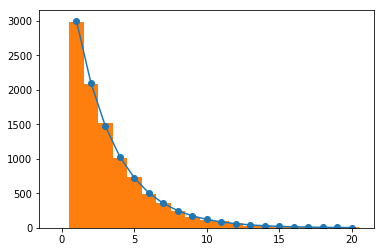In :
factorial(5)

Out:
array(120.)
In :
def poisson(x, rate):
result = numpy.exp(-rate) * (rate**x)
result /= scipy.special.factorial(x)
return result
print(poisson(numpy.array([1, 3, 9]), 10))

n_samples = 10000
x = numpy.linspace(0, 20, 21)
pyplot.plot(x, n_samples * poisson(x, 5), "o-")
pyplot.hist(numpy.random.poisson(5, size=n_samples), bins=range(0,22), align="left")
pyplot.show()

[0.000454   0.00756665 0.12511004]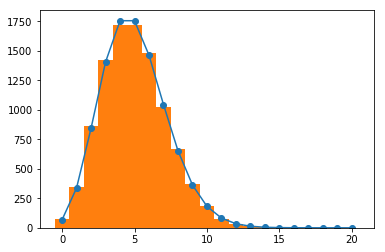In :
poisson_samples = numpy.random.poisson(10, size=10000)
print(numpy.mean(poisson_samples))

9.9873

In :
print(numpy.var(poisson_samples))

10.124738709999999

In :
len([ s for s in poisson_samples if s > 10 - numpy.sqrt(10) and s < 10 + numpy.sqrt(10) ])

Out:
7322
In :
std_dev = numpy.sqrt(10)
len([ s for s in poisson_samples if s > 10 - 2 * std_dev and s < 10 + 2*std_dev ])

Out:
9604

Now let's look at the normal or Gaussian distribution.

In :
def normal(x, mean, variance):
result = 1.0 / (numpy.sqrt(2 * numpy.pi * variance))
result *= numpy.exp( - (x - mean)**2 / (2 * variance))
return result
normal(0.5, 0, 1)

Out:
0.3520653267642995

Plotting this function over a linear space of 100 points between -5 and 5 looks good.

In :
x = numpy.linspace(-5, 5, 100)
y = normal(x, 0, 1)
pyplot.plot(x, y)
pyplot.show()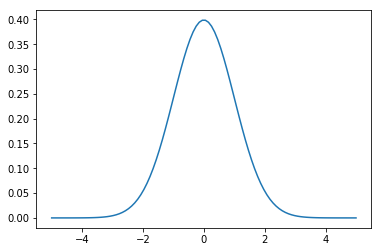We can also generate a large number of samples and plot an empirical distribution.

In :
normal_samples = numpy.random.normal(0, 1, size=10000)
normal_samples[0:10]

Out:
array([ 1.08496757,  0.0048767 ,  0.94218285,  0.21632084,  1.00697706,
-1.30270995, -2.52164597, -0.22192926,  0.09314268,  0.38271804])
In :
pyplot.hist(normal_samples, bins=numpy.linspace(-5, 5, 100))
pyplot.show()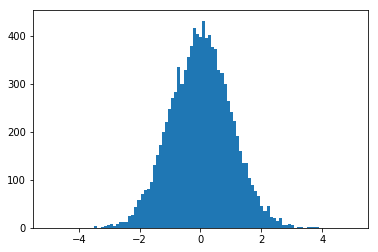But putting these together will be more challenging than before. Here's what happens if I just multiply the probability function by the sample size like we did for the previous two:

In :
## x and y haven't changed, but I'm repeating them here so we remember what they are
x = numpy.linspace(-5, 5, 100)
y = normal(x, 0, 1)
pyplot.plot(x, 10000 * y)
pyplot.hist(normal_samples, bins=x)
pyplot.show()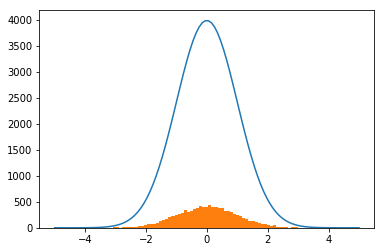Why is the blue line so far above the orange histogram? The difference is that the normal probability function is a probability density for a continuous variable. We actually want the probability of a sample falling within a bin of width 0.1. That probability is pretty close to $10000 \cdot 0.1 \cdot \mathcal{N}(X|\mu, \sigma^2)$

In :
## x and y haven't changed, but I'm repeating them here so we remember what they are
x = numpy.linspace(-5, 5, 100)
y = normal(x, 0, 1)
pyplot.plot(x, 10000 * ((5 - -5)/100) * y)
pyplot.hist(normal_samples, bins=x)
pyplot.show()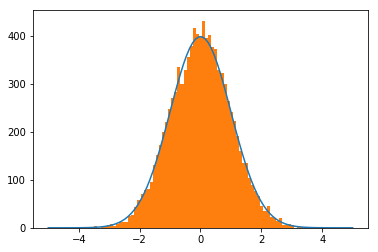The relationship between the standard deviation of normally distributed data and the probability of being outside a certain range is very stable. So much so that it has a name: the 68-95-99.7 rule. About 68% of samples should be within one standard deviation. Since I asked for normal samples with mean 0 and variance 1, the standard deviation should also be 1. Did this work for my 10000 samples?

In :
len([ s for s in normal_samples if s > -1 and s < 1 ])

Out:
6883

About 95% should be within two standard deviations:

In :
len([ s for s in normal_samples if s > -2 and s < 2 ])

Out:
9571

And about 99.7% should be within three standard deviations:

In :
len([ s for s in normal_samples if s > -3 and s < 3 ])

Out:
9975